# Digital Electronics - Boolean Algebra and Logic Simplification

## Why Digital Electronics Boolean Algebra and Logic Simplification?

In this section you can learn and practice Digital Electronics Questions based on "Boolean Algebra and Logic Simplification" and improve your skills in order to face the interview, competitive examination and various entrance test (CAT, GATE, GRE, MAT, Bank Exam, Railway Exam etc.) with full confidence.

## Where can I get Digital Electronics Boolean Algebra and Logic Simplification questions and answers with explanation?

IndiaBIX provides you lots of fully solved Digital Electronics (Boolean Algebra and Logic Simplification) questions and answers with Explanation. Solved examples with detailed answer description, explanation are given and it would be easy to understand. All students, freshers can download Digital Electronics Boolean Algebra and Logic Simplification quiz questions with answers as PDF files and eBooks.

## Where can I get Digital Electronics Boolean Algebra and Logic Simplification Interview Questions and Answers (objective type, multiple choice)?

Here you can find objective type Digital Electronics Boolean Algebra and Logic Simplification questions and answers for interview and entrance examination. Multiple choice and true or false type questions are also provided.

## How to solve Digital Electronics Boolean Algebra and Logic Simplification problems?

You can easily solve all kind of Digital Electronics questions based on Boolean Algebra and Logic Simplification by practicing the objective type exercises given below, also get shortcut methods to solve Digital Electronics Boolean Algebra and Logic Simplification problems.

### Exercise :: Boolean Algebra and Logic Simplification - General Questions

1.

Convert the following SOP expression to an equivalent POS expression.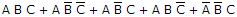A.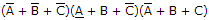B.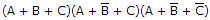C.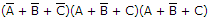D.Explanation:

No answer description available for this question. Let us discuss.

2.

Determine the values of A, B, C, and D that make the sum term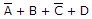equal to zero.

 A. A = 1, B = 0, C = 0, D = 0 B. A = 1, B = 0, C = 1, D = 0 C. A = 0, B = 1, C = 0, D = 0 D. A = 1, B = 0, C = 1, D = 1

Explanation:

No answer description available for this question. Let us discuss.

3.

Which of the following expressions is in the sum-of-products (SOP) form?

 A. (A + B)(C + D) B. (A)B(CD) C. AB(CD) D. AB + CD

Explanation:

No answer description available for this question. Let us discuss.

4.

Derive the Boolean expression for the logic circuit shown below: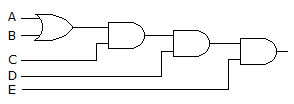A.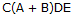B.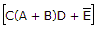C.D.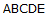Explanation:

No answer description available for this question. Let us discuss.

5.

From the truth table below, determine the standard SOP expression.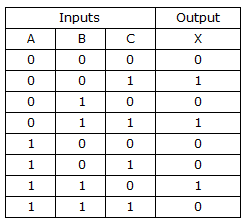A.B.C.D.